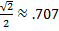# Coterminal Angles

Coterminal Angles are angles in standard position that have the same Initial Side and the same Terminal side. For example 45°, 405° and -315° are coterminal angles because all three angles have the same initial side (the x axis) and they share a same terminal side.There are two methods that can be used to determine if two or more angles are coterminal. The first one is to sketch the angles and determine if the terminal side is the same as per the figure above.

The second option is to determine mathematically if they are coterminal by subtracting 360 until a number between 0 and positive 360 can be reached. If the angle is negative add 360 until a number between 0 and positive 360 is reached.

405 - 360 = 45 therefore 405° and 45° are coterminal
-315 + 360 = 45 therefore -315° and 45° are coterminal

In trigonometry coterminal angles have the same trigonometric values. For instance, the sine of 45° istherefore the sine of 405° and -315° are also.

 Related Links: Math Trigonometry Functions of Large and Negative Angles Inverse Trigonometric Functions

To link to this Coterminal Angles page, copy the following code to your site: